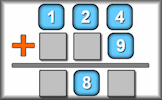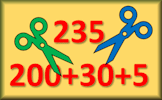# Number Systems

$$\mathbb N$$: The set of natural or counting numbers 0, 1, 2, 3, etc. Some say this set does not include zero.

$$\mathbb Z$$: The set of integers. This set is the same as the set of natural numbers but includes the negative whole numbers.

$$\mathbb Q$$: The set of rational numbers. These are the numbers that can be expressed as a proper fraction or one integer divided by another.

$$\mathbb R$$: The set of real numbers. That is probably all the numbers you know unless you have studied the topic of Complex Numbers.

$$\mathbb R$$
$$\mathbb Q$$
$$\mathbb Z$$
$$\mathbb N$$
$$\text{one million}$$
$$\frac34$$
$$\cos 40^o$$
$$\pi$$
$$\sin 30^o$$
$$-\sqrt9$$
$$\frac{57}{9}$$
$$\frac23$$
$$\sqrt2$$
$$1.\dot4\dot2$$
$$\frac12\div\frac12$$
$$7$$
$$-2.479315...$$
$$1.3$$
$$1\div6$$

#### Nine DigitsArrange the given digits one to nine to make three numbers such that two of them add up to the third. This is a great puzzle for practicing standard pen and paper methods of three digit number addition and subtraction.

Drag the numbers into the correct sets as shown in the Venn diagram above. When you have finished click on the 'Check' button above.

If you make any mistakes don't forget to do your corrections! You can have as many attempts as you want to get the right answer.

There is a solution and ad-free version of this activity available to those who have a Transum Subscription.

There are other related activities on the Transum Mathematics website to support your understanding of number.

#### Partition CluesPartition numbers in different ways according to the clues given. The higher levels are quite hard! So far this activity has been accessed 198 times and 57 Transum Trophies have been awarded for completing it.

Strange But True,

Monday, August 5, 2019

"Most natural numbers are very, very large!"

Do you have any comments? It is always useful to receive feedback and helps make this free resource even more useful for those learning Mathematics anywhere in the world. Click here to enter your comments.For All: